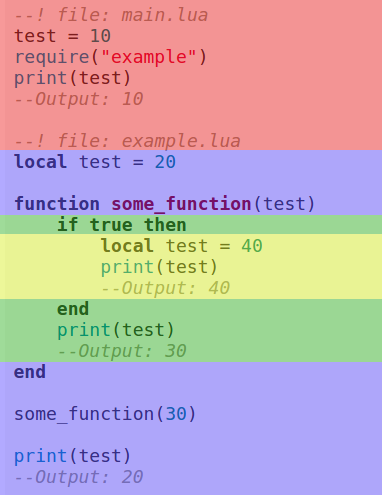# Chapter 9 - Multiple files and scope

## Multiple files

With multiple files, our code will look more organized, and easier to navigate. Create a new file called `example.lua`. Make sure it's in the same directory as `main.lua`.

Inside this file, create a variable. I will put `--! file:` at the top of the codeblock to make clear in what file you have to put the code.

``````--! file: example.lua
test = 20``````

Now in `main.lua`, put `print(test)`. When you run the game, you'll see that `test` equals `nil`. This is because we have to load the file first. We do this with `require`, by passing the filename as string as first argument.

``````--! file: main.lua
--Leave out the .lua
require("example")
print(test)``````

We don't add the `".lua"` in the filename, because Lua does this for us.

You can also put the file in a subdirectory, but in that case make sure to include the whole path.

``````--Use . instead of /
require("path.to.example")``````

Now when you print `test`, after we loaded `example.lua`, you should see it says 20.

`test` in this case is what we call a global variable. It's a variable that we can use everywhere in our project. The opposite of a global variable, is a local variable. You create a local variable by writing `local` in front of the variable name.

``````--! file: example.lua
local test = 20``````

Run the game to see test is once again `nil`. This is because of its scope.

## Scope

Local variables are limited to their scope. In the case of `test`, the scope is the file `example.lua`. This means that `test` can be used everywhere inside that file, but not in other files.

If we were to create a local variable inside a block, like a function, if-statement or for-loop, then that would be the variable's scope.

``````--! file: example.lua
if true then
local test = 20
end

print(test)
--Output: nil``````

`test` is `nil`, because we print it outside of its scope.

Parameters of functions are like local variables. Only existing inside the function.

To really understand how scope works, take a look at the following code:

``````--! file: main.lua
test = 10
require("example")
print(test)
--Output: 10``````
``````--! file: example.lua
local test = 20

function some_function(test)
if true then
local test = 40
print(test)
--Output: 40
end
print(test)
--Output: 30
end

some_function(30)

print(test)
--Output: 20``````

If you run the game, it should print: 40, 30, 20, 10. Let's take a look at this code step by step.

The first print is inside the if-statement, and it's 40. After the if-statement we print `test` again, and now it's 30, which is what we passed as argument. The parameter `test` was not affected by the `test` inside the if-statement. Inside the if-statement the local variable took priority over the parameter.

Outside of the function we also print `test`. This time it's 20. The `test` created a the start of `example.lua` was not affected by the `test` inside the function.

Lastly we print `test` in `main.lua`, and it's 10. The global variable was not affected by the local variables inside `example.lua`.

I made a visualization of the scope of each `test` to make it even more clear:When creating a local variable, you don't have to assign a value right away.

``````local test
test = 20``````

## Returning a value

Like functions, files can return a value. If we put `return 10` at the end of `example.lua`, and inside `main.lua` we do `print(require("example"))`, you will see that it prints 10.

## TL;DR

With `require` we can load other lua-files. When you create a variable you can use it in all files. Unless you create a local variable, which is limited to its scope. Local variables do not affect variables with the same name outside of their scope.